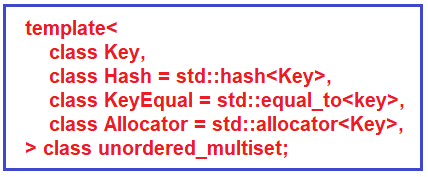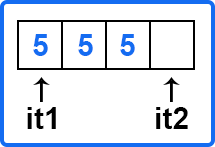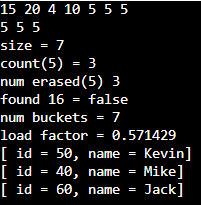# Unordered MultiSet in C++

## Unordered MultiSet in C++ with Examples

In this article, I am going to discuss Unordered Multiset in C++ with Examples. Please read our previous article, where we discussed Unordered Set in C++ with Examples. In our previous article, we had seen an ordered set. If you have not read that article, we would highly recommend you read that first.

##### Unordered MultiSet in C++:
1. It is an associative container that contains a set of non-unique objects of type key.
2. For using an unordered multiset, you have to include the ‘unordered_set’ header file.
3. It takes constant time for search, removal, and insertion operations.
4. It is implemented as Hash Table.Here we have a hash function and we need to provide it when we are defining a custom class. We want to store objects of custom class into our unordered set because STL will not know how to create a hash from the object of your custom class. It will not know how to compare them for equality. So, you will need to define the equal to (==) operator. And you would need to define a hash function where you will take two objects of your class and then create the hash. Instead of unique, it allows non-unique objects so you can have multiple objects of the same key that is you can insert multiple instances of the same key just like a normal multiset.

For unordered set and unordered multiset, we simply include the ‘unordered_set’ header file and for ordered set and multiset, we include the ‘set’ header file. Search, removal, and insertion operations take constant time because of hash table implementation. But the worst case can be that long chains are present in a particular bucket. That can create trouble and that can increase the time if your hash function is not good. Also, when you rehash something let us say you have defined 10 buckets only but you have inserted 1000 elements. So, each bucket will have a large number of elements even if your hash function is good. Each bucket will have 100 on average. So, this is a very bad load factor. So, you will need to rehash those things.

##### Unordered Multiset Functions in C++:
1. Size () – This function is used to find the number of elements present in the multiset container. This will treat each instance of a key distinctly for example 5 is inserted 3 times so it will count 3 times in the multiset but in a normal set, It would be inserted just once so it will be unique.
2. Equal (=) – it is used to assign multiset.
3. Clear () – it will remove all the elements from the multiset container.
4. Count (), find () – In count, you will pass a key and it will return you the number of occurrences of that element there. For example, count (5) will return 3 if there are 3 occurrences of 5. The find function will return an iterator to the element if it is present. For example, suppose we have a multiset m, m.find (3) so it will return the iterator to 3. In multiset, if we have duplicate values then the iterator will be on the lower bound. The lower bound means ‘>=’ and the upper bound is ‘<’. It’s generally used to check whether the element is present or not. We will compare the find function with unordered_multiset.end(). If the key is not present then it will return the end () iterator.
5. Equal_range () – We know that here we can have multiple instances of a particular key so we have a function called equal range. It will return a range. What is a range? A range is a pair of beginning and end. This range will contain two iterators it1 and it2. So, it will figure out all the elements that are matching with the particular key.

Let us say five is occurring three times so you can iterate through this complete range using this function. So it1 will be pointing to one of them and it t2 will point to one element past that.You can run a loop from it1 to it2 till the iterator is not equal to t2, keep on iterating and you can print or whatever you want to do with that you can do that.

1. Empty () – it will check and return whether the multiset is empty or not.
2. Insert () and erase () – we will see these functions in detail.
3. Bucket_count (), load_factor () – The underlying algorithm is a hash table underlying data structure so it will have bucket count (number of buckets). load factor is how the hash table is loaded and there are many more such things like you can also find the bucket of a particular key and many more things which you will generally not need but if you are interested you can explore more on these.

So, let us see insert an erase function in detail.

##### Insert Unordered Multiset Functions in C++:
1. Insert (Key key): It will not check for duplicity of the element and it will return an iterator to the inserted element
2. Insert (iterator pos1, iterator pos2): Here, we can pass two iterator positions. Let us say you have a vector of elements, V = {_ _ _ x _ _ _ y _ _ _ _ }; You want to insert from position x to position y (excluding y) and these are iterator positions. It is like range [pos1, pos2). So, the close interval at pos1 and open interval at pos2. This function will not return anything.
3. Insert (initializer_list l): Similarly, we can pass the initializer list. You can think of it as in the above example, if we are inserting from pos1 to pos2, it will be an initializer list or you can say a subset of the vector. So, you will pass that initializer list and it will add all the elements to the multiset. It will also not return anything. We will see examples of all of these functions.
##### Erase Unordered Multiset Functions in C++:
1. erase (iterator pos): It removes the element at the given position and returns the iterator following the last element removed.
2. erase (iterator pos1, iterator pos2): As we have seen in the insert function, we can also pass two positions in this function so that all the elements between these positions will be removed. So pos1 will be included and pos2 will be excluded. It also returns the iterator following the last element removed.
3. erase (key): If you do not know the position but you know the key you can pass the key and it will remove that key. In this signature, we have passed the iterator to the key but here we are passing the key itself. If any key is present in the multiset, it will remove all the elements with the key values. It will return the number of elements removed.

Now let’s see the complete program.

##### Example to understand Unordered Multiset in C++:
```#include <iostream>
#include <functional>
#include <unordered_set>
using namespace std;

class Student{
public:
int id;
string name;

bool operator==(const Student& s) const{
return (this->id == s.id && this->name == s.name);
}

void print_student() const {
cout << "[ id = " << id << ", name = " << name << "]\n";
}
};

class StudentHashFunction{
public:
size_t operator()(const Student& s) const{
return (hash<int>{}(s.id) + hash<string>{}(s.name));
}
};

int main(){

unordered_multiset<int> us = {5, 10, 4, 20, 5, 5, 15};
for(int x: us)
cout << x << " ";
cout << endl;

auto its = us.equal_range(5);
for(auto it = its.first; it != its.second; ++it)
cout << *it << " ";
cout << endl;

cout << "size = " << us.size() << endl;
cout << "count(5) = " << us.count(5) << endl;
cout << "num erased(5) " << us.erase(5) << endl;
cout << boolalpha << "found 16 = " << (us.find(16) != us.end()) << endl;
cout << "num buckets = " << us.bucket_count() << endl;